# SOCR EduMaterials ModelerActivities MixtureModel 1

## SOCR Modeler Activities - SOCR Mixture Model Fitting Activity

This is a SOCR Activity that demonstrates random sampling and fitting of mixture models to data

### SOCR Mixture-Model Distribution

The 1D SOCR mixture-model distribution enables the user to specify the number of mixture Normal distributions and their parameters (means and standard deviations). This applet demonstrates how unimodal-distributions come together as building-blocks to form the backbone of many complex processes. In addition, this applet allows computing probability and critical values for these mixture distributions, and enables inference on such complicated processes. Extensive demonstrations of mixture modeling in 1D, 2D and 3D are available on the SOCR EM Mixture Modeling page. The figure below shows one such example of a tri-modal mixture of 4 Normal distributions.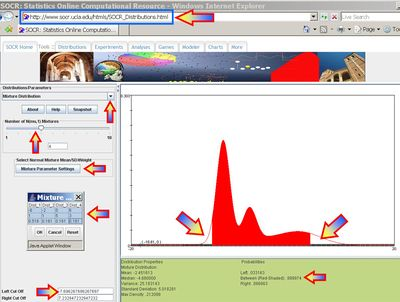### Data Generation

You typically have investigator-acquired data that you need to fit a model to. In this case we will generate the data by randomly sampling using the SOCR resource. Go to the SOCR Modeler and select the Data Generation tab from the right panel.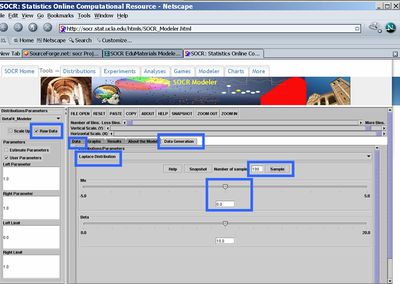• Now, click the Raw Data check-box in the left panel, select Laplace Distribution (or any other distribution you want to sample from), choose the sample-size to be 100 (keep the center, Mu ($$\mu=0$$)) and click Sample. Then go to the Data tab, in the right panel. There you should see the 100 random Laplace observations stored as a column vector.
• Next, go back to the Data Generation tab from the right panel and change the center of the Laplace distribution (set Mu=20, say). Click Sample again and you will see the list of randomly generated data in the Data tab expand to 200 (as you just sampled another set of 100 random Laplace observations).

### Exploratory Data Analysis (EDA)

Go to the Data tab and select all observations in the data column (use CTR-A, or mouse-copy). Then open another web browser and go to SOCR Charts. Choose HistogramChartDemo2, say, clear the default data (Data tab) and paste (CTR-V or mouse paste-in) in the first column the 200 observations that you sampled in the SOCR Modeler Data Generator (above). Then you need to map the values - go to the Mapping tab, select the first column, where you pasted the data (C1), and click XValue. This will move the C1 column label from the left bin to the bottom-right bin. Finally, click Update Chart, on the top, and go to the Graph tab to see your histogram of the 200 (bimodal) Laplace observations. Notice, that you can change the width of the histogram bin to clearly see the bi-modality of the distribution of these 200 measurements. Of course, this is due to the fact that we sampled from two distinct Laplace distributions, one with mean of zero and the second with mean of 20.0.### Model Fitting

Now go back to the SOCR Modeler browser (where you did the data sampling). Choose Mixed-Model-Fit from the drop-down list in the left panel.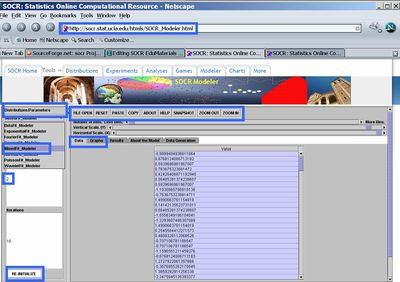• We will now try to fit a 2-component mixture of Gaussian (Normal) distributions to this Bimodal Laplace distribution (of the generated sample). You may need to click the Re-Initialize button a few times. The Expectation-Maximization algorithm used to estimate the mixture distribution parameters is unstable and will produce somewhat different results for different initial conditions. Hence, you may need to re-initialize the algorithm a few times until a visually satisfactory result is obtained.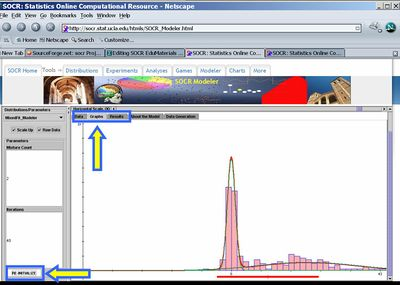• Notice the quantitative results of this mixture model fitting protocol (in the Results panel). Recall that we sampled 100 observations from Laplace distribution with mean of zero (not Normal Gaussian, which we could also have done and the fit would have been much better, of course) and then another 100 observations from Laplace distribution with mean = 20.0. In this case, the reported estimates of the means of the two Gaussian mixtures are 0 and 22 (pretty close to the original/theoretical means). We could have also fit in a mixture of 3 (or more) Gaussian mixture components, if we had a reason to believe that the data distribution is tri- (or higher-)modal, and therefore, requires a multi-modal mixture fit.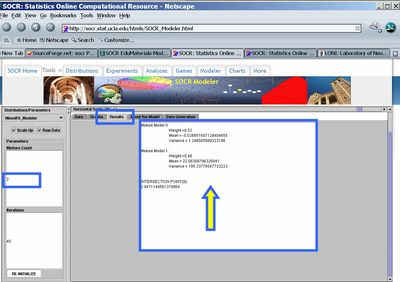• There are statistical tests added to assess:
• how statistically significant are the mean values of any pair of Gaussian models $$\left \{N(\mu_1,\sigma_1^2), N(\mu_2,\sigma_2^2)\right\}$$ in the Mixture Distributions. Normal Z tests are use for this assessment $$\left \{ Z_o=\frac{\mu_1-\mu_2}{\sqrt{\frac{\sigma_1^2}{N_1}+ \frac{\sigma_2^2}{N_2}}} \sim N(0,1^2)\right \}$$.
• And how good the overall mixture model fir tot he data is, using the Kolmogorov-Smirnoff Test.

### Caution

You may need to properly set the values of the sliders on the top of your Graph tab, in the right panel, so that you can see the entire graph of the histogram and the models fit to the data. Also, the random data you generate and the EM algorithm are stochastic and you can not expect to get exactly the same results and charts as reported in this SOCR activity. Everyone that tries to replicate these steps will obtain different results, however, the principles we demonstrate here are indeed robust.